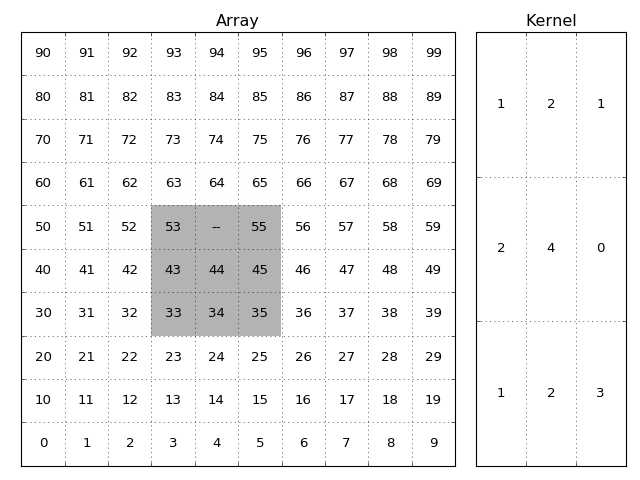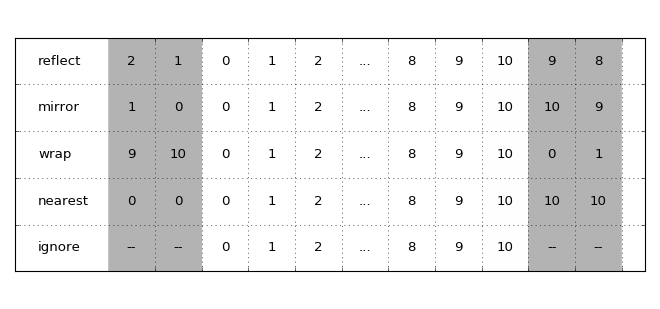`numbamisc` includes several filter (convolution) functions:

• `sum_filter`
• `average_filter`

as well as the following rank filters:

• `median_filter`
• `median_filter_weighted`
• `min_filter`
• `max_filter`

The main difference to the ones in other libraries is that these handle masked arrays. However this imposes some restrictions as well:

• The kernel for `sum_filter` and `average_filter` must not contain mixed positive and negative values.
• The functions are slower because they cannot exploit common filter optimizations.

Note

The filters have an artificial limit of 5 dimensions. If you need higher dimensional support you can alter the `numbamisc/_filters.py` file. There should be a line `fobj.write(generate(maxndim=5))`, the `maxndim` value restricts the maximum dimension of the functions.

Each filter function accepts a `mask` argument. But for some data types it is not necessary to specify it explicitly. For example if the `data` argument is a

• `numpy.ma.MaskedArray`
• `astropy.nddata.NDData`

object then it’s mask will be used by default!

It’s possible to override this by explicitly passing in a `mask`.

## Kernels¶

The kernel is an array of weights that is applied to each local neighborhood to calculate the resulting filtered value. The kernel has different interpretations depending on the kind of filter.

• For `sum_filter` and `average_filter` it represents an array of weights.
• For `median_filter`, `min_filter` and `max_filter` it represents a boolean condition if the values should be taken into account.
• For `median_filter_weighted` it represents integer weights.The image and kernel. The grey shadowed part of the array are the elements used when calculating the result of the (x=5, y=4) item. The `--` represents a masked value.

The value of the output element will be:

`1*53 + 0(2*'--') + 1*55 + 2*43 + 4*44 + 0*45 + 1*33 + 2*34 + 3*35`

divided by the sum of the valid kernel elements:

`1+0+1+2+4+0+1+2+3`

and in case of `sum_filter` thereafter multiplied by the total sum of kernel elements.

`1+2+1+2+4+0+1+2+3`

To ease using the kernel there are some shortcuts if the kernel is simple, if the `kernel` argument is an:

• `int`, then it’s assumed that this integer gives the shape in all dimensions and the kernel should contain ones. For example when the data has 3 dimensions and `kernel=4` is used then internally the kernel will be converted to `np.ones((4, 4, 4))`.
• `tuple`, then this will be used as `shape` argument for a kernel containing ones: `np.ones(kernel)`.
• `astropy.convolution.Kernel`, then it’s array attribute is used.

Otherwise an explicit `numpy.array` object is expected.

## Border handling¶

These filters accept the following `border` arguments:

• `ignore` (default)
• `reflect`
• `mirror`
• `nearest`
• `wrap`

Except for `ignore` (which ignores values outside the array) these are used to specify how the array is padded if the `kernel` goes outside the array grid.How the options affect how the array is padded. The grey areas indicate the padded values.

## NaN handling¶

Each filter has the option to also treat `NaN` values as masked. Just pass `ignore_nan=True` to the filter function.